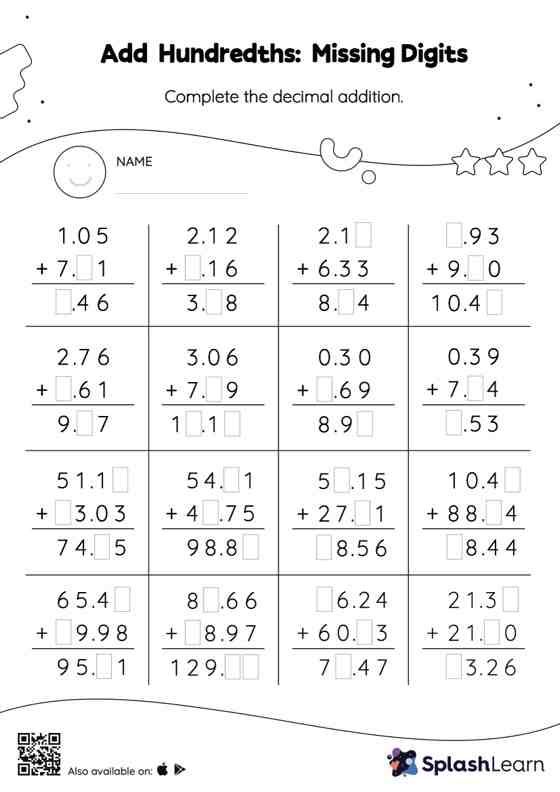# Add Hundredths: Missing Digits Worksheet

Home > Add Hundredths: Missing DigitsStudents demonstrate their problem-solving ability as they work on this add hundredths worksheet. When adding decimals on this worksheet, students align the decimal points and use zero as a placeholder. Then to find the missing number in the add hundredths worksheet, students then apply the relationship between addition and subtraction. This worksheet is about practicing the column method in which numbers are written one on top of another. In this method, students use their place value understanding to solve the problems.